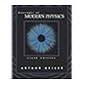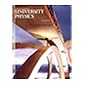Normal view MARC view ISBD view

# Fundamentals of physics / David Halliday, Robert Resnick, with the assistance of W. Farrell Edwards, John Merrill.

Material type:TextPublisher: New York : Wiley, c1981Edition: 2d ed.Description: xv, 816,  p. : ill. ; 27 cm.ISBN: 0471033634 ; 9780471033639.DDC classification: 530 Online resources: WorldCat details | E-book Fulltext
Contents:
Table of contents Measurement -- Vectors -- Motion in one dimension -- Motion in a plane -- Particle dynamics--I -- Particle dynamics--II -- Work and energy -- The conservation of energy -- The dynamics of systems of particles -- Collisions -- Rotational kinematics -- Rotational dynamics -- Equilibrium of rigid bodies -- Oscillations -- Gravitation -- Fluid mechanics -- Waves in elastic media -- Sound waves -- Temperature -- Heat and the first law of thermodynamics -- Kinetic theory of gases -- Entropy and the second law of thermodynamics -- Charge and matter -- The electric field -- Gauss's law -- Electric potential -- Capacitors and dielectrics -- Current and resistance -- Electromotive force and circuits -- The magnetic field -- Ampre's law -- Faraday's law of induction -- Inductance -- Magnetic properties of matter -- Electromagnetic oscillations -- Alternating currents -- Maxwell's equations -- Electromagnetic waves -- Geometrical optics -- Interference -- Diffraction -- Light and quantum physics -- Waves and particles -- Appendices. A. The International System of Units (SI) -- B. Some fundamental constants of physics -- C. Some properties of the sun, the earth, and the moon -- D. Some properties of the planets -- E. The periodic table of the elements -- F. Conversion factors -- G. Mathematical symbols and the Greek alphabet -- H. Mathematical formulas -- I. Trigonometric functions -- J. Winners of the Nobel Prize in physics.
Summary: This text addresses the issue of building "bridges of reason", so that students may move from qualitative understanding of any given physics concept to making decisions about how to solve a problem involving that concept.
Tags from this library: No tags from this library for this title.Average rating: 0.0 (0 votes)
Item type Current location Collection Call number Copy number Status Date due Barcode Item holdsE-Book
E-book
Non-fiction 530 HAF 1981 (Browse shelf) Not For LoanText
Reserve Section
Non-fiction 530 HAF 1981 (Browse shelf) C-1 Not For Loan 5933
Total holds: 0
##### Browsing EWU Library Shelves , Shelving location: E-book Close shelf browser530.44 VEW 2000 Waves in dusty space plasmas / 530.8 BEP 1995 Principles of measurement systems / 530 BEC 2003 Concepts of modern physics / 530 HAF 1981 Fundamentals of physics / 530 YOU 2016 Sears and Zemansky's University physics / 531.6 ENE 2008 Energy and movement 531 PLT 1971 Theoretical mechanics for sixth forms

Includes index.

Vectors --
Motion in one dimension --
Motion in a plane --
Particle dynamics--I --
Particle dynamics--II --
Work and energy --
The conservation of energy --
The dynamics of systems of particles --
Collisions --
Rotational kinematics --
Rotational dynamics --
Equilibrium of rigid bodies --
Oscillations --
Gravitation --
Fluid mechanics --
Waves in elastic media --
Sound waves --
Temperature --
Heat and the first law of thermodynamics --
Kinetic theory of gases --
Entropy and the second law of thermodynamics --
Charge and matter --
The electric field --
Gauss's law --
Electric potential --
Capacitors and dielectrics --
Current and resistance --
Electromotive force and circuits --
The magnetic field --
Ampre's law --
Inductance --
Magnetic properties of matter --
Electromagnetic oscillations --
Alternating currents --
Maxwell's equations --
Electromagnetic waves --
Geometrical optics --
Interference --
Diffraction --
Light and quantum physics --
Waves and particles --
Appendices. A. The International System of Units (SI) --
B. Some fundamental constants of physics --
C. Some properties of the sun, the earth, and the moon --
D. Some properties of the planets --
E. The periodic table of the elements --
F. Conversion factors --
G. Mathematical symbols and the Greek alphabet --
H. Mathematical formulas --
I. Trigonometric functions --
J. Winners of the Nobel Prize in physics.

This text addresses the issue of building "bridges of reason", so that students may move from qualitative understanding of any given physics concept to making decisions about how to solve a problem involving that concept.

Applied Physics & Electronics

There are no comments for this item.